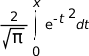erfc(), erfcf(), erfcl()

Complementary error function

Synopsis:

#include <math.h>

double erfc ( double x );

float erfcf ( float x );

long double erfcl( long double x );

Arguments:

x
The number for which you want to compute the complementary error function.

Library:

libm

Use the -l m option to qcc to link against this library.

Description:

The erfc(), erfcf(), and erfcl() functions calculate the complementary error function of x (i.e., the result of the error function, erf(), subtracted from 1.0). This is useful because the error function isn't very accurate when x is large.

The erf() function computes:This equality is true: erfc(-x) = 2 - erfc(x)

Returns:

The value of the error function, or NAN if x is NAN. For a correct value that would cause an underflow, these functions return 0.0.

Note: If an error occurs, these functions return 0, but this is also a valid mathematical result. If you want to check for errors, set errno to 0, call the function, and then check errno again. These functions don't change errno if no errors occurred.

Classification:

Safety:
Cancellation point No
Interrupt handler No
Signal handler No